Trial ends in
JoVE Science Education
General Chemistry

This content is Free Access.

Subtitles
Introduction to Titration
##### Science Education (General Chemistry)
Determining the Density of a Solid and Liquid
##### Science Education (General Chemistry)
Common Lab Glassware and Uses

# Introduction to Titration

### Overview

Source: Laboratory of Dr. Yee Nee Tan — Agency for Science, Technology, and Research

Titration is a common technique used to quantitatively determine the unknown concentration of an identified analyte.1-4 It is also called volumetric analysis, as the measurement of volumes is critical in titration. There are many types of titrations based on the types of reactions they exploit. The most common types are acid-base titrations and redox titrations.5-11

In a typical titration process, a standard solution of titrant in a burette is gradually applied to react with an analyte with an unknown concentration in an Erlenmeyer flask. For acid-base titration, a pH indicator is usually added in the analyte solution to indicate the endpoint of titration.12 Instead of adding pH indicators, pH can also be monitored using a pH meter during a titration process and the endpoint is determined graphically from a pH titration curve. The volume of titrant recorded at the endpoint can be used to calculate the concentration of the analyte based on the reaction stoichiometry.

For the acid-base titration presented in this video, the titrant is a standardized sodium hydroxide solution and the analyte is domestic vinegar. Vinegar is an acidic liquid that is frequently used as a culinary condiment or flavorings. Vinegar mainly consists of acetic acid (CH3COOH) and water. The acetic acid content of commercial vinegar can vary widely and the goal of this experiment is to determine the acetic acid content of commercial vinegar by titration.

### Principles

The determination of acetic acid in vinegar is based on the principle of an acid-base titration method. The reaction between NaOH and CH3COOH is shown in Equation 1:

CH3COOH(aq) + NaOH(aq) → H2O(l) + NaCH3CO2(aq) (1)

The standardized NaOH solution is progressively added to the vinegar with unknown acetic acid concentration until the end point is reached. During the acid-base titration, the pH can be plotted as a function of the volume of the titrant added. The inflection point on the curve, the point at which there is a stoichiometric equal amount of acid and base in a solution, is called the equivalence point. Most acids and bases are colorless, with no visible reaction occurring at the equivalence point. To observe when the equivalence point has been reached, a pH indicator is added. The endpoint is not the equivalence point but a point at which the pH indicator changes color. It is important to select an appropriate pH indicator so that the end point is as close to the equivalence point of titration as possible.

At the end point of this reaction, the conjugate base NaCH3CO2 is slightly basic. Phenolphthalein indicator has a working pH range of 8.3–10.0, which is colorless in acidic solution and magenta above pH 8.2. Therefore, phenolphthalein is a preferred indicator as it will change from colorless to pink at this condition. When performing the experiment, it is best to keep the concentration of pH indicator low because pH indicators themselves are usually weak acids that react with base.

The volume of standardized NaOH solution added at end point can then be used to calculate the molar concentrations of acetic acid based on the stoichiometry of the above equation. In this experiment, the titrant NaOH is a strong alkaline and the analyte acetic acid is a weak acid.

Before performing the experiment, it is important to consider the hygroscopic nature of NaOH. This property requires its solution to be standardized with a stable primary standard such as potassium hydrogen phthalate (KHC8H4O4). The exact molar concentration of NaOH solution can then be accurately determined after standardization. The reaction between the primary acid standard and NaOH is shown in Equation 2:

KHC8H4O4(aq) + NaOH(aq) → H2O(l) + NaKC8H4O4(aq) (2)

A detailed step-by-step titration protocol is presented in the following section.

### Procedure

1. Standardization of NaOH with Potassium Hydrogenphthalate (KHC8H4O4)

1. To begin, the titrant, sodium hydroxide, must be standardized. Prepare a stock NaOH solution by dissolving about 4 g of NaOH pellet in 100 mL of deionized water. Note that NaOH is a hazardous chemical which is corrosive to skin and irritant to eye, be cautious and wear proper personal protection equipment (PPE) to avoid skin or eye contact.
2. Make a 1:10 dilution of the sodium hydroxide solution by adding 25 mL of the stock sodium hydroxide solution to a 500-mL bottle. Sodium hydroxide absorbs carbon dioxide. It is important to prevent this by making sure to use boiled, deionized water, an oven-dried bottle, and to cap the bottle quickly. Make the solution up to 250 mL with the deionized water and shake to mix.
3. Dry 4–5 g of the primary standard acid, KHC8H4O4 at 110 °C for 4 h in a drying oven and then cool the solid in a desiccator for 1 h.
4. Dissolve about 4 g of dried KHC8H4O4 in 250 mL of deionized water. Record the mass accurately. Calculate the molar concentration of the KHC8H4O4 solution.
5. Pipette 25 mL of KHC8H4O4 into a clean and dry Erlenmeyer flask. Add 2 drops of phenolphthalein, and swirl gently to mix well. Note that phenolphthalein is toxic and irritant, be cautious to avoid skin or eye contact.
6. Clean a 50-mL burette and a funnel thoroughly with detergent and water. Flush the burette with water and rinse 3x with deionized water. Rinse the burette with the diluted NaOH solution 3x, making sure that the NaOH wets the entire inner surface and drain the waste through the tip. Mount the washed burette on a ringstand with a clamp and ensure that it stands vertically.
7. Fill the clean burette with the diluted NaOH solution. It should be noted that the amount of the diluted NaOH needs not be exactly at the zero mark but should be within the scale and sufficient for at least one titration. Air bubbles may affect the accuracy of volume reading. Carefully check the burette for air bubbles, and gently tap the burette to free them and open the stopcock to let a few mL of titrant to flow through and at the same time releasing any trapped air. Read the volume by viewing the bottom of the meniscus after 10 s. Record this initial volume. Pay attention to the significant figures of the reading. Record the value to two decimal places in mL.
8. Place the Erlenmeyer flask containing potassium hydrogen phthalate (KHC8H4O4) under the burette and adjust the height of the burette properly. Titrate the KHC8H4O4 solution by slowly adding NaOH solution in 1–2 mL increments using one hand to control the flow rate by adjusting the stopcock, and the other swirling the flask.
9. When close to the endpoint, begin adding the titrant drop by drop. The endpoint is reached when the solution turns a faint, persistent pink color. Record the final volume of the diluted NaOH in the burette.
10. Repeat the titration at least twice more to obtain consistent data. Calculate the molar concentration of the diluted NaOH solution.

2. Titration of Vinegar with Standardized Sodium Hydroxide Solution

1. The sodium hydroxide solution is now standardized and can be used as a titrant to analyze vinegar. To reduce the pungent aroma of vinegar, dilute 10 mL of the vinegar solution to be tested in a 1:10 ration to a total volume of 100 mL.
2. Pipette 25 mL of analyte, to a clean and dry Erlenmeyer flask (noted as VA). Add 2 drops of phenolphthalein.
3. Fill the burette with the standardized NaOH solution from the first part of the Procedure. Record the initial volume of titrant (V1).
4. Progressively add the standardized NaOH solution to the vinegar. When the volume of titrant approaches the expected value, adjust the stopcock to add the titrant drop by drop. Continue to swirl the flask with one hand and keep the other hand ready to close the stopcock. Once the analyte solution changes to light pink color, swirl for a few seconds to see whether the color will fade. If the color persists, the titration reaches the end point. Record the final volume of titrant (V1'). If the solution color fades, add one more drop of titrant. Wash the bottom tip of the burette using the wash bottle. Collect the washed mixture and watch the color change of the analyte solution. Continue the titration till the end point. Record the amount of titrant needed (Vt1 = V1' V1).
5. Repeat the titration at least twice until three concordant values that are within 0.1 mL of one another is obtained (Vt2 and Vt3).
6. Calculate the mean value of titrant volume using the three values obtained in three different titrations: Vt = (Vt1 + Vt2 + Vt3)/3. The molar concentration of acetic acid in vinegar can be thus calculated using Equation 3.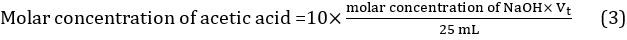Titration is a commonly applied method of quantitative chemical analysis used to determine the unknown concentration of a solution. A typical titration is based on a reaction between a titrant and an analyte. The titrant of known concentration is gradually added to a precise volume of an unknown analyte until the reaction reaches an endpoint.

At the endpoint, the moles of titrant and analyte are equal. By manipulating the equation relating volume and concentration, the concentration of analyte can be deduced.

This video will illustrate the principles behind titration, present a protocol to determine the amount of acetic acid in commercial vinegar, and finally explore some common applications of the method.

Titrations are classified based on the type of reaction carried out. For example, redox titrations make use of an oxidation-reduction exchange between reactants which involves the transfer of electrons from one reactant to another. Complexometric titrations rely on the formation of a largely undissociated complex. However, acid-base titrations, which exploit the neutralization of an acid with a base, are one of the most widely studied. To determine the concentration of acid in an analyte, a base, such as sodium hydroxide, is used. Sodium hydroxide is hygroscopic, that is, it has the property of absorbing moisture from the atmosphere. Before it can be used as a titrant, its exact concentration in solution must be standardized.

To do this, it is first titrated with the primary standard, potassium hydrogen phthalate. A primary standard should be pure, stable, non-hygroscopic, and have a high molecular weight. Because the amount of hydronium ions contributed by the primary standard is known to a high degree of accuracy, it is used to determine the exact concentration of the hydroxide ions in the titrant. During an acid-base titration, the pH can be plotted as a function of the volume of the titrant added. The inflection point on the curve, the point at which there is a stoichiometric equal amount of acid and base in a solution, is called the equivalence point.

Most acids and bases are colorless, with no visible reaction occurring at the equivalence point. To observe when the equivalence point has been reached, a pH indicator is added. This is a pH sensitive dye that changes color in different pH environments. Its important to note that endpoint is not equal to the equivalence point, but indicates when a particular pH value has been reached. For example, phenolphthalein changes color around a pH of 8 and is commonly used as an indicator for acid-base titrations with an equivalence point around pH 7. While an accurate indicator for the titration is one that changes color as close to the equivalence point as possible, the titration curve has a steep slope around the equivalence point, leading to an acceptable level of error. At the equivalence point, the moles of base added are equal to the moles of acid initially present. An equation that utilizes the molarity and volume of each component can be used. With the other three values known, the acid concentration can be calculated. Now that you understand the principles behind the procedure, lets take a look at an actual protocol to determine the percent acetic acid in a commercial vinegar sample by reacting it with a standardized sodium hydroxide solution.

Typically, a rough estimate titration is performed to approximate where the endpoint will be. To begin, the titrant, sodium hydroxide, must be standardized. First, dissolve roughly 4 g of sodium hydroxide into 100 mL of deionized water. Make a 1:10 dilution by adding 25 mL of this stock sodium hydroxide solution to a glass container. Bring the total volume to 250 mL with deionized water and shake to mix. As sodium hydroxide absorbs carbon dioxide, it is important to use boiled, deionized water and an oven-dried bottle, and to cap the bottle quickly.

Calculate the approximate molar concentration of sodium hydroxide. Then, weigh out 5 g of the standard acid, potassium hydrogen phthalate, and place it in a drying oven. Once dried, allow the solid to cool to room temperature in a desiccator.

Weigh out 4 g of the dried potassium hydrogen phthalate to a high degree of precision, and dissolve in 250 mL of deionized water. Calculate the molar concentration of the potassium hydrogen phthalate solution.

Using a volumetric pipette, transfer 25 mL of the potassium hydrogen phthalate solution into a clean, dry Erlenmeyer flask. Add 2 drops of phenolphthalein pH indicator. Gently swirl the flask to mix. Flush a clean 50-mL burette with water and rinse at least three times with deionized water. Following this, rinse again with the diluted sodium hydroxide solution three times, making sure that the sodium hydroxide wets the entire inner surface. Mount the washed burette on a ringstand with a clamp and ensure that it stands vertically.

Fill the burette with the diluted sodium hydroxide solution. Air bubbles can affect the accuracy of volumetric readings. Gently tap the burette to free any air bubbles present, and open the stopcock to allow a few mL of titrant to flow through to release any trapped air. Read the volume of sodium hydroxide, at the bottom of the meniscus.

Place the flask containing potassium hydrogen phthalate under the burette. Add the titrant from the burette in 1–2 mL increments using one hand to control the flow rate by adjusting the stopcock, and the other swirling the flask.

When close to the endpoint, begin adding the titrant drop by drop. The endpoint is reached when the solution turns a faint, persistent pink color. Record the volume in the burette.

Repeat the titration at least two more times for consistent data and calculate the molar concentration of the diluted sodium hydroxide solution used as shown in the text protocol.

The sodium hydroxide solution is now standardized and can be used as a titrant to analyze vinegar. To reduce the pungent aroma, dilute 10 mL to a total volume of 100 mL.

Pipette 25 mL of the diluted vinegar into an Erlenmeyer flask, and add 2 drops of phenolphthalein. Fill the burette with the standardized sodium hydroxide solution and record the initial volume. Similar to the previous titration, slowly add the titrant to the analyte in the flask while swirling until the solution turns a light pink color, and record the final volume of sodium hydroxide used.

In this experiment, the titration was performed in triplicate and the mean volume of sodium hydroxide dispensed to neutralize the acetic acid in vinegar was calculated. The concentration and volume of base was used to elucidate the moles of acetic acid in the vinegar. The volume and molar mass were then used to calculate the concentration. It was determined that the vinegar had a molarity of 0.7388. Converting to percent, it was 4.23% acetic acid by volume.

Titrations are robust and easily customizable methods commonly applied in research, industry, and healthcare.

Scientists often use the measure of dissolved oxygen in freshwater bodies as an indicator of overall health that ecosystem. This is done by a redox titration. Unlike acid-base neutralizations, these titrations are based on a reduction-oxidation reaction between the analyte and the titrant. Dissolved oxygen in the water sample is reduced with chemicals in a reaction that results in the production of iodine. The amount of iodine produced and thus the level of dissolved oxygen can be determined by titration using a starch indicator. Glucose in urine can be indicative of a pathological condition like diabetes. A test to quantify urine glucose level, called Benedict's Method, is another example of the importance of titration; in this case, in healthcare. In this titrimetric procedure, sugars from urine are first reacted with an alkali resulting in the formation of enediols with powerful reducing properties. These reduce copper two ions in Benedict's reagent to copper one, in a colorimetric reaction that correlates with the initial concentration of glucose present in the urine sample.

You've just watched JoVE's introduction to titration. You should now be familiar with the principles behind this method, know how to perform an acid-base titration, and appreciate some of the ways it is being applied in research and industry.

As always, thanks for watching!

### Results

 Unit Trial 1 Trial 2 Trial 3 Volume of diluted vinegar acid (VA) mL 25.00 Molar concentration of NaOH (cNaOH) mol/L 0.09928 Initial burette reading of NaOH mL 0.10 0. 05 1.20 Final burette reading of NaOH mL 18.75 18.60 19.80 Volume of NaOH dispensed mL 18.65 18.55 18.60 Mean volume of NaOH dispensed (Vt) mL 18.60

Table 1. Titration results.

Sample calculations:

Mass of KC8H5O4 = 4.0754 g

Molar mass of KC8H5O4 = 204.22 g/mol

Number of moles of KC8H5O4 in 25.00 mL standard solution =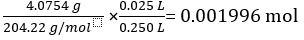According to Equation 2,

Concentration of the diluted NaOH solution =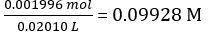Moles of NaOH dispensed = concentration of NaOH × mean volume of NaOH dispensed = 0.09928 mol/L × 18.60 mL = 1.847 × 10-3 mol

According to Equation 1,

Number of moles of CH3COOH in 25.00 mL of diluted vinegar = 1.847 × 10-3 mol

Concentration of diluted vinegar =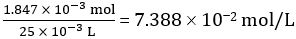Hence concentration of undiluted vinegar = 10 × 7.388 102 mol/L = 0.7388 mol/L

The above steps are presented to illustrate the calculation procedure; we can simply apply Equation 3 to obtain the concentration of undiluted vinegar in one step.

Therefore 1.000 L of undiluted vinegar contains 0.7388 mol of CH3COOH.

Volume of CH3COOH=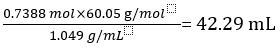Volume percent of vinegar =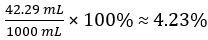### Applications and Summary

Titration is an important chemical method that is frequently applied in current chemistry research. For example, acid base titration is applied to determine amine or hydroxyl value of a sample. The amine value is defined as the number of milligrams of KOH equivalent to the amine content in one gram of sample. To determine the hydroxyl value, the analyte is first acetylated using acetic anhydride then titrated with KOH. The mass in milligrams of KOH then corresponds to hydroxyl groups in one gram of sample.13 Another example is the Winkler test, a specific type of redox titration used to determine the concentration of dissolved oxygen in water for water quality studies. Dissolved oxygen is reduced using manganese(II) sulfate, which then reacts with potassium iodide to produce iodine. Since the iodine released is directly proportional to the oxygen content, the oxygen concentration is determined by titrating iodine with thiosulfate using a starch indicator.14

Besides applications in basic chemical research, titration has also been widely adopted in industrial and everyday use. In biodiesel industry, waste vegetable oil (WVO) must first be neutralized to remove free fatty acids that would normally react to make undesired soap. A portion of WVO is titrated with a base to determine the sample acidity, so the rest of the batch could be properly neutralized.15 Benedict's method, a test for quantification of urine glucose level, is another example showing the importance of titration in healthcare. In this titration, cupric ions are reduced to cuprous ions by glucose, which then react with potassium thiocyanate to form a white precipitate, indicating the endpoint.16

### Get cutting-edge science videos from JoVE sent straight to your inbox every month.X